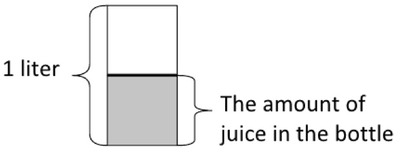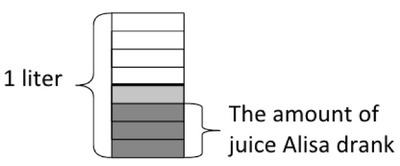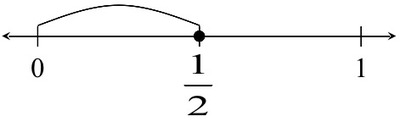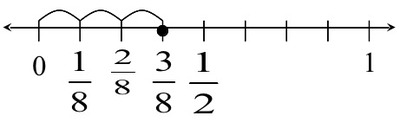# Drinking Juice

Alignments to Content Standards: 5.NF.B.6

Alisa had $\frac{1}{2}$ a liter of juice in a bottle. She drank $\frac{3}{4}$ of the juice that was in the bottle. How many liters of juice did she drink?

## IM Commentary

This is the second problem in a series of three tasks involving fraction multiplication that can be solved with pictures or number lines. The first, 5.NF Running to school, does not require that the unit fractions that comprise $\frac{3}{4}$ be subdivided in order to find $\frac{1}{3}$ of $\frac{3}{4}$. This task does require students to subdivide the unit fractions that comprise $\frac{1}{2}$ in order to find $\frac{3}{4}$ of $\frac{1}{2}$. The third task, 5.NF Half of a Recipe, also requires subdivision and involves multiplying a fraction and a mixed number.

Solutions which involve (sub)dividing a quantity into equal parts in order to find a fraction of the quantity should be emphasized, especially at first. While multiplication of fractions (or decimals) yields appropriate solutions which should be recognized as such, students need to see and be able to produce the types of solutions represented here as well.

The context here involves volume. While the picture can be drawn to look like liquid in a bottle, the number line representation is more abstract relative to the context. This helps transition students to a more abstract understanding of fraction multiplication.

Teachers should be aware that a student who attends to the context only in a superficial way or attempts to solve simply by matching operations to keywords is likely to misread the problem as one involving the difference of $\frac34$ and $\frac12$ and obtain a nonsense answer.

## Solutions

Solution: Drawing a Picture

The first picture represents the $\frac{1}{2}$ liter of juice in the bottle.The second picture represents $\frac{3}{4}$ of the juice in the bottle, which is what Alisa drank.Because each half liter has been divided into 4 equal pieces, the whole liter has been divided into 8 equal pieces. Since what Alisa drank is represented by 3 of these pieces, she drank $\frac{3}{8}$ liters of juice.

Solution: Using a Number Line

First plot a point at $\frac{1}{2}$, that represents the amount of juice in the bottle.Divide the segment between 0 and $\frac{1}{2}$ into four equal lengths so that we can plot $\frac{3}{4}$ of $\frac{1}{2}$. Also divide the segment between $\frac{1}{2}$ and 1 into four equal lengths so we can see that the segment between 0 and 1 is divided into 8 equal lengths. Labeling the segments in eighths, we can see $\frac{3}{4}$ of $\frac{1}{2}$ is $\frac{3}{8}$.Because each half liter has been divided into 4 equal pieces, the whole liter has been divided into 8 equal pieces. Since what Alisa drank is represented by 3 of these pieces, she drank $\frac{3}{8}$ liters of juice.

Solution: Computing a Product

If students understand that finding a fraction of some quantity, that is scaling that quantity by a fraction, corresponds to multiplication then we can proceed by calculation. Since we want to know what $\frac{3}{4}$ of $\frac{1}{2}$ liter is, we can multiply: \begin{equation} \frac{3}{4} \times \frac{1}{2} = \frac{3 \times 1}{4 \times 2} = \frac{3}{8} \end{equation} Alisa drank $\frac{3}{8}$ liters of juice.

Solution: Using Decimal Numbers

A student may recognize that $\frac{1}{2} = 0.5$ and $\frac{3}{4} = 0.75$ and proceed by multiplying decimal numbers:

$$0.75 \times 0.5 = 0.375$$

So that Alisa drank $0.375$ liters of juice.

Solution: Solution Using Cuisenaire Rods

The problem can be solved using Cuisenaire rods. A student might notice that we are working with $\frac12$ and $\frac34$, and so might use the red (which is 2 white rods long) and the purple rod (which is 4 white rods long) so that she could represent fourths and halves. However, since the question asks for $\frac34$ of $\frac12$ liter, the student cannot break $\frac12$ of the purple rod (which is equivalent to a red rod) into fourths. So the student would need to use a brown rod (which is 8 white rods long) to represent the whole liter. The brown rod is equivalent to 2 purple rods, so a purple rod represents $\frac12$ liter. The student can then use the white rod to show fourths of the purple rod (which represents the amount of juice in the bottle). $\frac14$ of the purple rod is equivalent to $\frac18$ of the brown rod. $\frac34$ of the purple rod is the same as $\frac38$ of the Brown rod.

So Alisha drank $\frac38$ liters of juice.

Alternatively, since we need to find $\frac34$ of the amount of juice, a student might use the purple rod (which is 4 white rods long) to represent the amount of juice in the bottle so that she could represent fourths of the juice. But the juice in the bottle is only half of a liter, so we need twice as much to represent the whole liter. Putting two purples together represents one whole liter, and we can see that $\frac34$ of $\frac12$ of the liter is $\frac38$ of the liter.